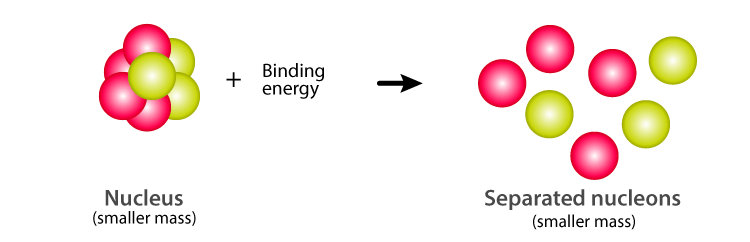Jet Set Go! All about Aeroplanes Jet Set Go! All about Aeroplanes

# Nuclear Binding Energy

During the 20th century, the popular Albert Einstein came up with the revolutionary theory, “the theory of relativity”. The theory explained that mass and energy are interconvertible; the mass can be converted into energy and vice-versa. This new dimension to physics helped to resolve plenty of unsolved problems and formed a forum for lot of new theories. One among them is the existence of Nuclear Binding energy. Thereby giving a clear insight into nuclear mass and inter-nuclei interactions.## Mass Defect and Binding Energy

An atom comprises a nucleus at the center and electrons revolving around it in an orbital fashion. Nuclei constitute of Protons and Neutrons, Combined called nucleons. Thus, we expect that mass of the nucleus will be the same as the sum of individual masses of neutrons and protons. But it is not true. The total mass of the nucleus(mnuc) is less than the sum of individual masses of neutrons and protons which in fact constitutes it. This difference in the mass is called mass defect given by,

$$\begin{array}{l}\Delta m=Zm_{p}+(A-Z)m_{n}-m_{nuc}\end{array}$$

Where,

Zmp is the total mass of the protons.

(A-Z)mn is the total mass of the neutrons.

mnuc is the mass of the nucleus.

According to Einstein’s theory of relativity, The mass-energy is equivalent. That is the mass of a system measures the total energy of the system. Given by the famous equation E=mc2. Hence, it is implied that the total energy of the nucleus is less than the sum of the energies of individual protons and neutrons(nucleons). This implies that when the nucleus disintegrates into constituent nucleons releasing some energy in the form of heat energy. (Exothermic reaction). The energy emitted here is mathematically expressed using

$$\begin{array}{l}E=\left (\Delta m\right )c^{2}\end{array}$$

Now imagine the situation of breaking the nucleus. To attain this, a certain amount of energy is put into the system. The amount of energy required to achieve this is called nuclear binding energy. Thus, we can define nuclear binding energy as,

“The minimum energy required to separate nucleons into its constituent protons and neutrons.” and is given by-

$$\begin{array}{l}E_{b}=\left (\Delta m\right )c^{2}\end{array}$$

Thus, the difference in the mass is converted into Nuclear binding energy.

## How to calculate binding energy?

The nuclear binding energy can be calculated following the below-given steps:

• Once the mass defect is known, the nuclear binding energy can be calculated by converting that mass to energy using the formula
$$\begin{array}{l}E_{b}=\left (\Delta m\right)c^{2}\end{array}$$
.
• Make sure that the mass is in the units of kgs
• Once the energy obtained is known, it can be scaled into per-nucleon and per- mole quantities.

You may want to check out the following articles for a better understanding of nuclear binding energy.

### Binding Energy Calculation

1)Calculate the Binding Energy of the Deuteron. Give data: a mass of the deuteron is 1875.61MeV/c2 or 3.34359✕10-27 Kg.

Solution:

Given:

Mass of deuteron mD = 1875.61MeV/c2 or 3.34359✕10-27 Kg.

Atomic Mass number of Deuteron; A=2

The atomic number of Deuteron; Z=1

Mass defect Δm =?

Binding energy Eb = ?

Formula used:

Mass defect is given by

$$\begin{array}{l}\Delta m=Zm_{p}+(A-Z)m_{n}-m_{nuc}\end{array}$$

Binding energy is given by

$$\begin{array}{l}E_{b}=\left (\Delta m\right )c^{2}\end{array}$$

Calculation:

Mass defect is given by

$$\begin{array}{l}\Delta m=Zm_{p}+(A-Z)m_{n}-m_{nuc}\end{array}$$
. Here Z=1 and (A-Z)=1, mn =939.57MeV/c2 and mp =938.28 MeV/c2. Substituting the values we get-

Δm = mp + mn– mD

= 938.28 MeV/c2 + 939.57MeV/c2 – 1875.61MeV/c2

=2.24 MeV/c2

Thus, the mass defect is 2.24 MeV/c2

The binding energy of the Deuteron is thus given by

$$\begin{array}{l}E_{b}=\left (\Delta m\right )c^{2}\end{array}$$

= (2.24 MeV/c2)(c2)

=2.24 MeV

A minimum of 2.24 million electron volt energy is required to break Deuteron into Proton and Neutron. This is a very large value. However, the energy required to separate an electron from a hydrogen atom by overcoming electromagnetic force(Coulomb force) is approximately 10eV. This comparison clearly indicates the strength of the nuclear force. Although its range lies in multiple Femto meters, It is one of the strongest forces in nature.

Stay tuned with BYJU’S for more such interesting topics. Register to “BYJU’S-The Learning App” for the latest set of interactive engaging physics videos.

Test your knowledge on Nuclear Binding Energy